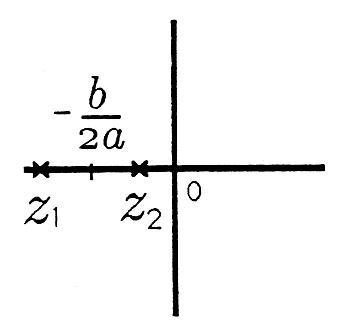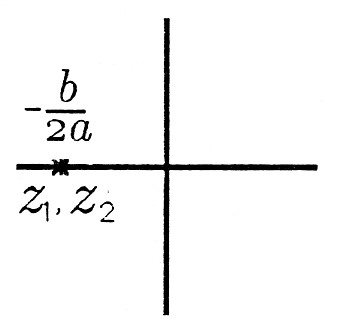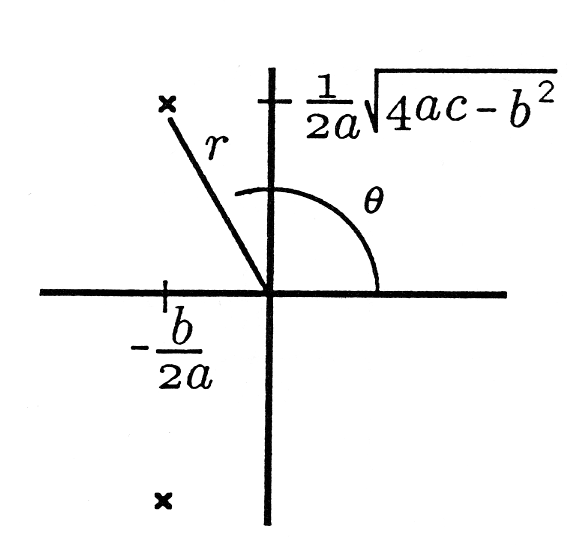# 2.3 Complex numbers: roots of quadratic equations

 Page 1 / 1

You probably first encountered complex numbers when you studied values of $z$ (called roots or zeros) for which the following equation is satisfied:

$a{z}^{2}+bz+c=0.$

For $a\ne 0$ (as we will assume), this equation may be written as

${z}^{2}+\frac{b}{a}z+\frac{c}{a}=0.$

Let's denote the second-degree polynomial on the left-hand side of this equation by $p\left(z\right)$ :

$p\left(z\right)={z}^{2}+\frac{b}{a}z+\frac{c}{a}.$

This is called a monic polynomial because the coefficient of the highest-power term $\left({z}^{2}\right)$ is 1. When looking for solutions to the quadratic equation ${z}^{2}+\frac{b}{a}z+$ $\frac{c}{a}=0$ , we are really looking for roots (or zeros) of the polynomial $p\left(z\right)$ . The fundamental theorem of algebra says that there are two such roots. When wehave found them, we may factor the polynomial $p\left(z\right)$ as follows:

$p\left(z\right)={z}^{2}+\frac{b}{a}z+\frac{c}{a}=\left(z-{z}_{1}\right)\left(z-{z}_{2}\right).$

In this equation, z 1 and z 2 are the roots we seek. The factored form $p\left(z\right)=$ $\left(z-{z}_{1}\right)\left(z-{z}_{2}\right)$ shows clearly that $p\left({z}_{1}\right)=p\left({z}_{2}\right)=0$ , meaning that the quadratic equation $p\left(z\right)=0$ is solved for $z={z}_{1}$ and $z={z}_{2}$ . In the process of factoring the polynomial $p\left(z\right)$ , we solve the quadratic equation and vice versa.

By equating the coefficients of ${z}^{2},{z}^{1}$ , and z 0 on the left-and right-hand sides of [link] , we find that the sum and the product of the roots ${z}_{1}$ and ${z}_{2}$ obey the equations

${z}_{1}+{z}_{2}=-\frac{b}{a}{z}_{1}{z}_{2}=\frac{c}{a}.$

You should always check your solutions with these equations.

Completing the Square. In order to solve the quadratic equation ${z}^{2}+\frac{b}{a}z+\frac{c}{a}=0$ (or, equivalently, to find the roots of the polynomial ${z}^{2}+$ $\frac{b}{a}z+\frac{c}{a}\right)$ , we “complete the square” on the left-hand side of [link] :

${\left(z+\frac{b}{2a}\right)}^{2}-{\left(\frac{b}{2a}\right)}^{2}+\frac{c}{a}=0.$

This equation may be rewritten as

${\left(z+\frac{b}{2a}\right)}^{2}={\left(\frac{1}{2a}\right)}^{2}\left({b}^{2}-4ac\right).$

We may take the square root of each side to find the solutions

${z}_{1,2}=-\frac{b}{2a}±\frac{1}{2a}\sqrt{{b}^{2}-4ac}.$

In the equation that defines the roots ${z}_{1}$ and ${z}_{2}$ , the term ${b}^{2}-4ac$ iscritical because it determines the nature of the solutions for ${z}_{1}$ and ${z}_{2}$ . In fact, we may define three classes of solutions depending on ${b}^{2}-4ac$ .

(i) Overdamped $\left({b}^{2}-4ac>0\right)$ . In this case, the roots ${z}_{1}$ and ${z}_{2}$ are

${z}_{1,2}=-\frac{b}{2a}±\frac{1}{2a}\sqrt{{b}^{2}-4ac}.$

These two roots are real, and they are located symmetrically about the point $-\frac{b}{2a}$ . When $b=0$ , they are located symmetrically about 0 at the points $±\frac{1}{2a}\sqrt{-4ac}$ . (In this case, $-4ac>0.$ ) Typical solutions are illustrated in [link] .Typical Roots in the Overdamped Case; (a) b / 2 a > 0 , 4 a c > 0 , (b) b / 2 a > 0 , 4 a c < 0 , and (c) b / 2 a = 0 , 4 a c < 0

(ii) Critically Damped $\left({b}^{2}-4ac=0\right)$ . In this case, the roots ${z}_{1}$ and ${z}_{2}$ are equal (we say they are repeated):

${z}_{1}={z}_{2}=-\frac{b}{2a}.$

These solutions are illustrated in [link] .Roots in the Critically Damped Case; (a) b / 2 a > 0 , and (b) b / 2 a < 0

(iii) Underdamped $\left({b}^{2}-4ac<0\right)$ . The underdamped case is, by far, the most fascinating case. When ${b}^{2}-4ac<0$ , then the square root in the solutions for ${z}_{1}$ and ${z}_{2}$ ( [link] ) produces an imaginary number. We may write ${b}^{2}-4ac$ as $-\left(4ac-{b}^{2}\right)$ and write ${z}_{1,2}$ as

${z}_{1,2}=-\frac{b}{2a}±\frac{1}{2a}\sqrt{-\left(4ac-{b}^{2}\right)}=-\frac{b}{2a}±j\frac{1}{2a}\sqrt{4ac-{b}^{2}}.$

These complex roots are illustrated in [link] . Note that the roots are

purely imaginary when $b=0$ , producing the result

${z}_{1,2}=±j\sqrt{\frac{c}{a}}.$Figure 1.12: Typical Roots in the Underdamped Case; (a) b / 2 a > 0 , ( b ) b / 2 a < 0 , and (c) b / 2 a = 0

In this underdamped case, the roots ${z}_{1}$ and ${z}_{2}$ are complex conjugates:

${z}_{2}={z}_{1}^{*}.$

Thus the polynomial $p\left(z\right)={z}^{2}+\frac{b}{a}z+\frac{c}{a}=\left(z-{z}_{1}\right)\left(z-{z}_{2}\right)$ also takes the form

$p\left(z\right)=\left(z-{z}_{1}\right)\left(z-{z}_{1}^{*}\right)={z}^{2}-2Re\left[{z}_{1}\right]z+{|{z}_{1}|}^{2}.$

$Re\left[{z}_{1}\right]$ and $|{z}_{1}{|}^{2}$ are related to the original coefficients of the polynomial as follows:

$2Re\left[{z}_{1}\right]=-\frac{b}{a}$
$|{z}_{1}{|}^{2}=\frac{c}{a}$

Always check these equations.

Let's explore these connections further by using the polar representations for ${z}_{1}$ and ${z}_{2}$ :

${z}_{1,2}=r{e}^{±j\theta }.$

Then [link] for the polynomial $p\left(z\right)$ may be written in the “standard form”

$p\left(z\right)=\left(z-r{e}^{j\theta }\right)\left(z-r{e}^{-j\theta }\right)={z}^{2}-2rcos\theta z+{r}^{2}.$

$2rcos\theta =-\frac{b}{a}{r}^{2}=\frac{c}{a}$

These equations may be used to locate ${z}_{1,2}=r{e}^{±j\theta }$

$r=\sqrt{\frac{c}{a}}\theta =±{cos}^{-1}\left(\frac{-b}{\sqrt{4ac}}\right).$

where we get a research paper on Nano chemistry....?
nanopartical of organic/inorganic / physical chemistry , pdf / thesis / review
Ali
what are the products of Nano chemistry?
There are lots of products of nano chemistry... Like nano coatings.....carbon fiber.. And lots of others..
learn
Even nanotechnology is pretty much all about chemistry... Its the chemistry on quantum or atomic level
learn
da
no nanotechnology is also a part of physics and maths it requires angle formulas and some pressure regarding concepts
Bhagvanji
hey
Giriraj
Preparation and Applications of Nanomaterial for Drug Delivery
revolt
da
Application of nanotechnology in medicine
what is variations in raman spectra for nanomaterials
ya I also want to know the raman spectra
Bhagvanji
I only see partial conversation and what's the question here!
what about nanotechnology for water purification
please someone correct me if I'm wrong but I think one can use nanoparticles, specially silver nanoparticles for water treatment.
Damian
yes that's correct
Professor
I think
Professor
Nasa has use it in the 60's, copper as water purification in the moon travel.
Alexandre
nanocopper obvius
Alexandre
what is the stm
is there industrial application of fullrenes. What is the method to prepare fullrene on large scale.?
Rafiq
industrial application...? mmm I think on the medical side as drug carrier, but you should go deeper on your research, I may be wrong
Damian
How we are making nano material?
what is a peer
What is meant by 'nano scale'?
What is STMs full form?
LITNING
scanning tunneling microscope
Sahil
how nano science is used for hydrophobicity
Santosh
Do u think that Graphene and Fullrene fiber can be used to make Air Plane body structure the lightest and strongest. Rafiq
Rafiq
what is differents between GO and RGO?
Mahi
what is simplest way to understand the applications of nano robots used to detect the cancer affected cell of human body.? How this robot is carried to required site of body cell.? what will be the carrier material and how can be detected that correct delivery of drug is done Rafiq
Rafiq
if virus is killing to make ARTIFICIAL DNA OF GRAPHENE FOR KILLED THE VIRUS .THIS IS OUR ASSUMPTION
Anam
analytical skills graphene is prepared to kill any type viruses .
Anam
Any one who tell me about Preparation and application of Nanomaterial for drug Delivery
Hafiz
what is Nano technology ?
write examples of Nano molecule?
Bob
The nanotechnology is as new science, to scale nanometric
brayan
nanotechnology is the study, desing, synthesis, manipulation and application of materials and functional systems through control of matter at nanoscale
Damian
Is there any normative that regulates the use of silver nanoparticles?
what king of growth are you checking .?
Renato
What fields keep nano created devices from performing or assimulating ? Magnetic fields ? Are do they assimilate ?
why we need to study biomolecules, molecular biology in nanotechnology?
?
Kyle
yes I'm doing my masters in nanotechnology, we are being studying all these domains as well..
why?
what school?
Kyle
biomolecules are e building blocks of every organics and inorganic materials.
Joe
Got questions? Join the online conversation and get instant answers!

#### Get Jobilize Job Search Mobile App in your pocket Now!By David CoreyBy IES PortalBy Dan ArielyBy OpenStaxBy David CoreyBy John GabrieliBy Richley CrapoBy OpenStaxBy Richley CrapoBy Yacoub Jayoghli